# How to Calculate Percentage

Go back to  'Percentages'

 1 Introduction to Percentage 2 How to Calculate Percentage? 3 Percentage Formula 4 Thinking out of the Box! 5 Percentage: Increase and Decrease with Solved Examples 6 Important Notes on Percentage 7 Practice Questions on Percentage 8 Maths Olympiad Sample Papers 9 Frequently Asked Questions (FAQs)

We at Cuemath believe that Math is a life skill. Our Math Experts focus on the “Why” behind the “What.” Students can explore from a huge range of interactive worksheets, visuals, simulations, practice tests, and more to understand a concept in depth.

Book a FREE trial class today! and experience Cuemath’s LIVE Online Class with your child.

## Introduction to Percentage

In Mathematics, we use numbers for comparisons.

Percentage is one of the ways to make comparisons.

Jack and Jolly got their report cards from school.Jack got 320 out of 400 whereas Jolly got 300 out of 360

Jack says that he has done better.

Do you agree with him?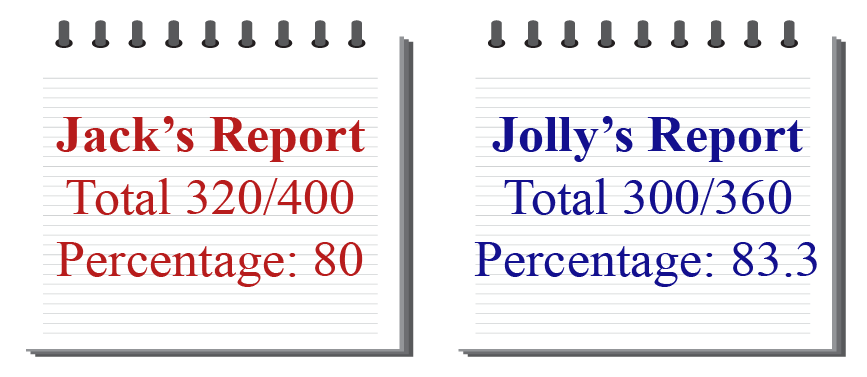Jack's mother says that they cannot decide this by just comparing the total marks obtained because the maximum marks are different for both of them.

Since Jolly's percentage is higher than Jack's percentage, this shows Jolly has done better.

Do you agree?

## How to Calculate Percentage?

### What is Percentage?

Percentages are fractions with denominator as 100

The word "percent" is derived from "per centum" which means "per hundred".

It is represented by %

### How to get Percentage?

Percent is another name for indicating hundredths.

Thus, 1% is one hundredth.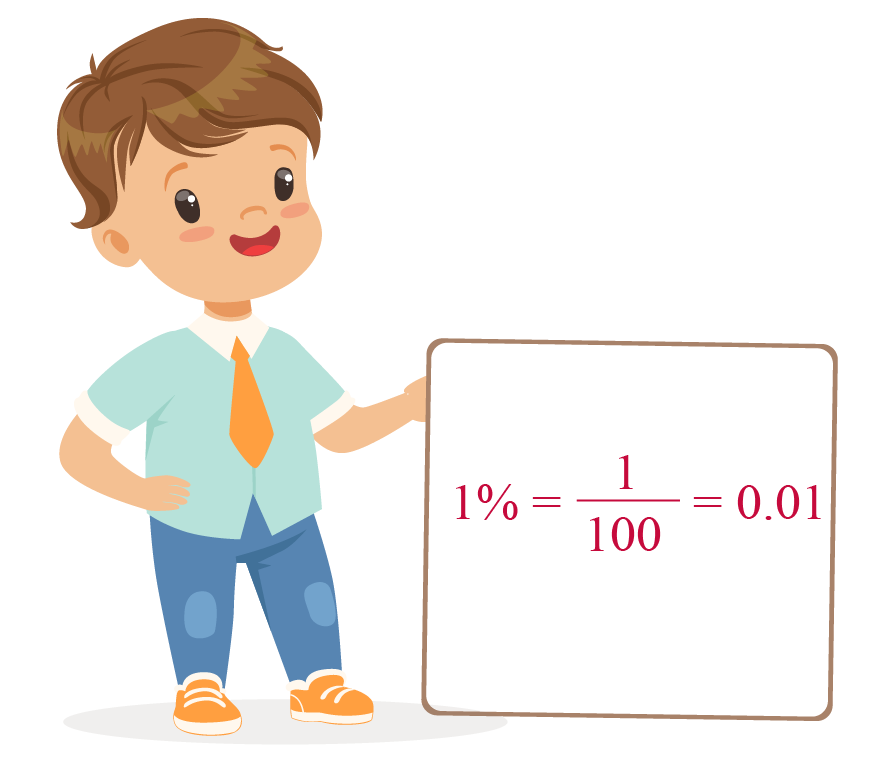Lovina bought tiles of three different colors for her house.

Look at the table below to see the details of this purchase.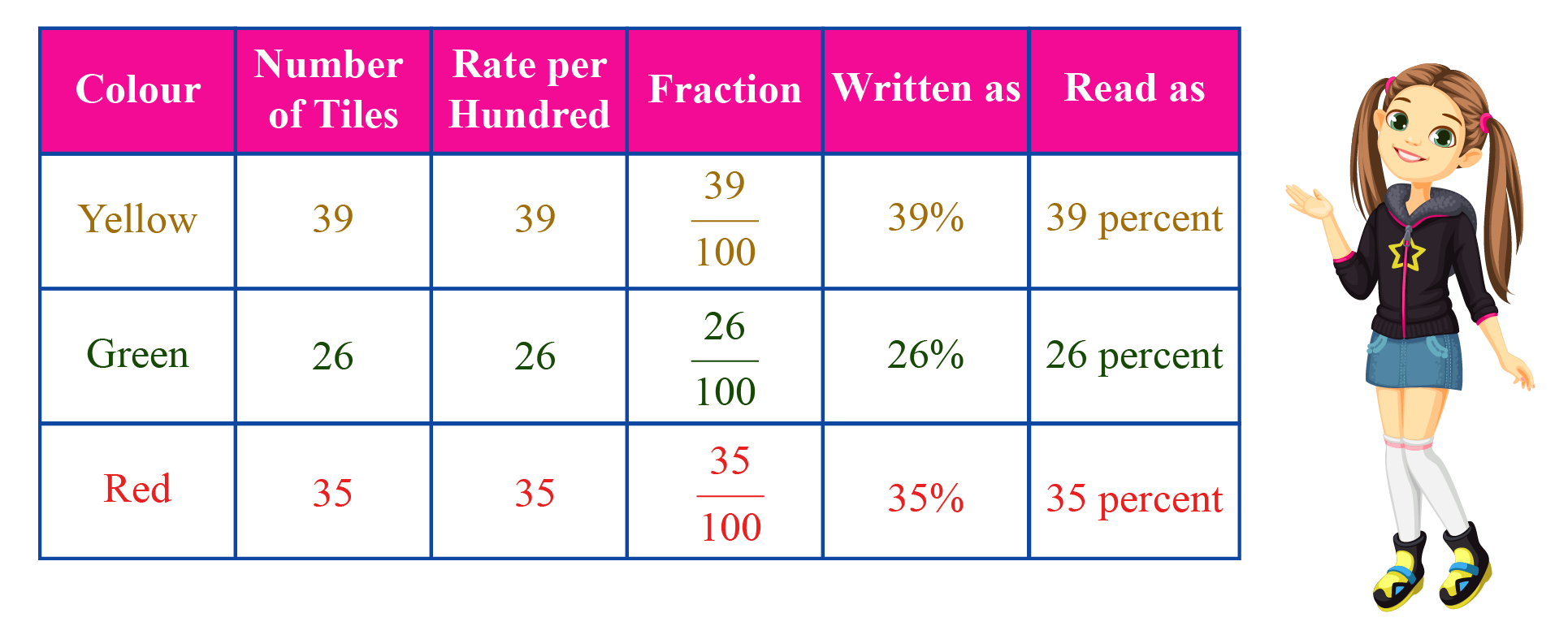Since the total number of items add up to 100, the percentages can be easily calculated.

What if the total number of items does not add up to 100?

In such cases, we convert fractions to equivalent fractions with denominator as 100

Let's look at the following example to see how this is done.

Emma has a bracelet with 20 beads in two colours.

The table below shows how to calculate the percentages of the red and blue beads.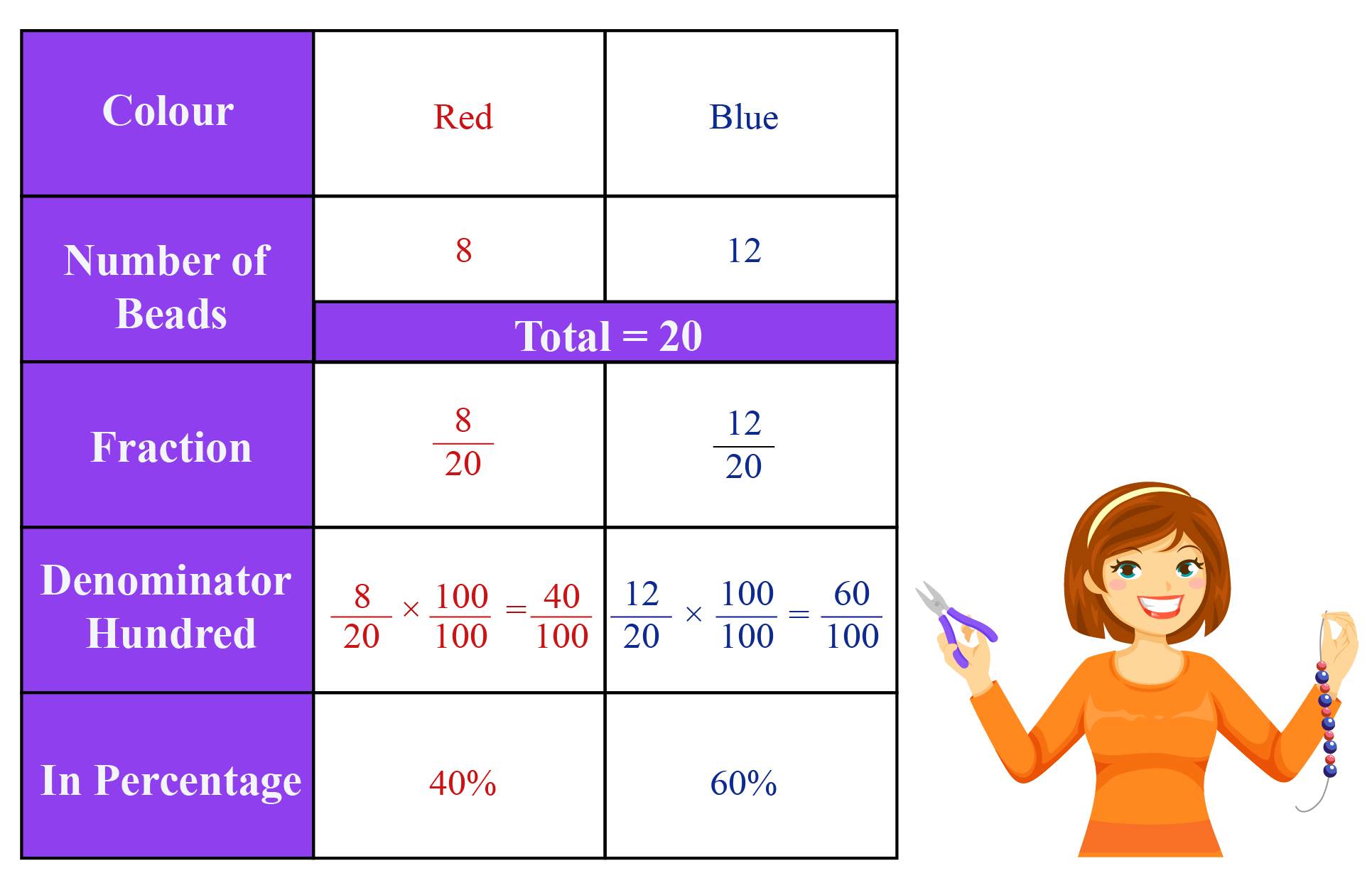Emma's sisters, Nora and Jenny, calculated the percentages as well, but in different ways.

Nora used the unitary method.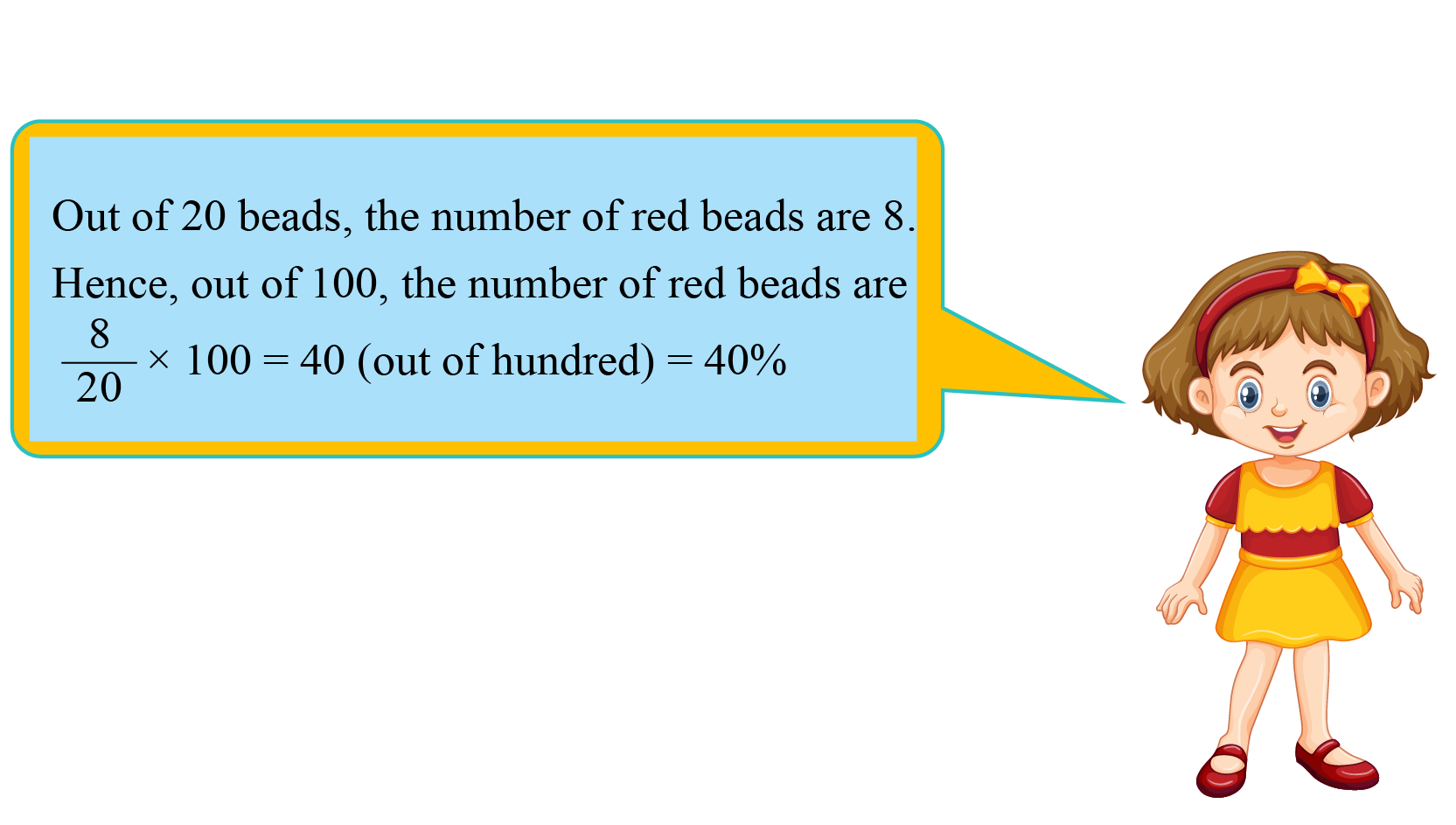Jenny converted the fraction \begin{align}\frac{8}{20}\end{align} into an equivalent fraction \begin{align}\frac{40}{100}\end{align} by multiplying the numerator and denominator with \begin{align}\frac{5}{5}\end{align}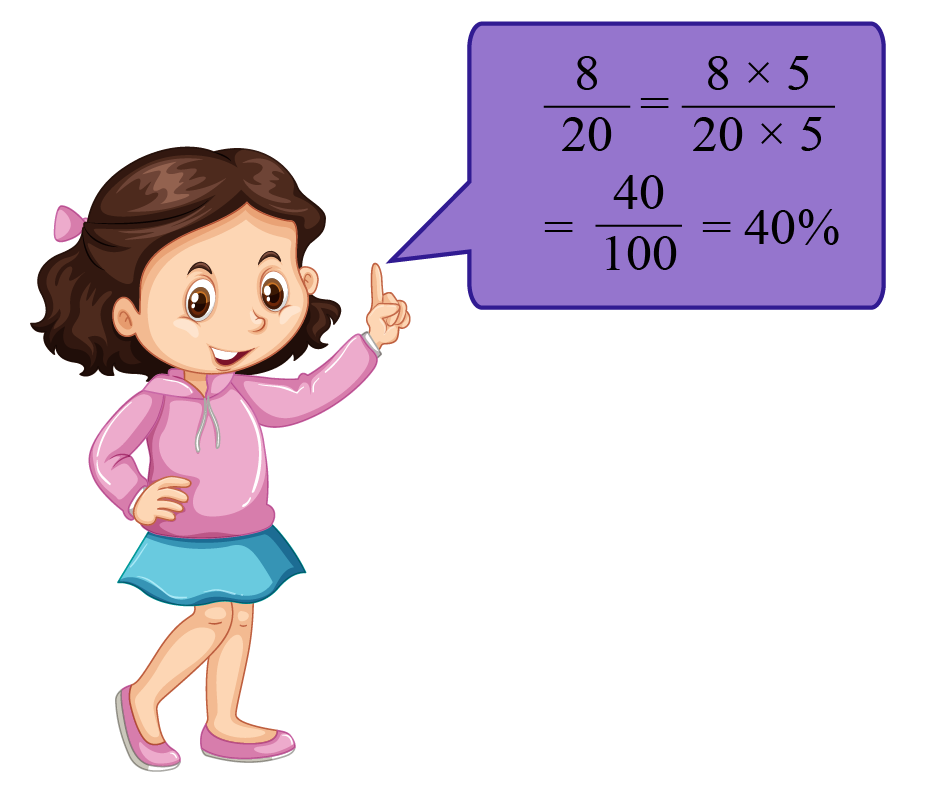You have now learned three different ways to calculate percentage when the total does not add up to 100

You can use the method you find most suitable to calculate percentages.

Let's experiment with some percentages in the simulation below.

Set a base amount and the percentage of the tip to be given.

You can observe the amount for the tip being calculated.

The percentage of the tip from the total amount is also visually depicted in the figure.

## Percentage Formula

Percentage formula is used to find the share of a whole in terms of 100.

Using this formula, you can represent a number as a fraction of 100.

 $$\text{Percentage}=\frac{\text{Value}}{\text{Total Value}} \times 100$$

### Calculate Percentage Difference

Sometimes we need to know the increase or decrease in some quantity as percentages also referred to as Percentage Change

For example, increase in population, decrease in poverty, and so on.

We have a formula to show the change in quantity as a percentage.

Percentage increase is given by the formula:

 \begin{align} \text{% Increase } \!\!=\! \frac{\text{Increased Amount}}{\text{Original Base}}\!\! \times\! 100\end{align}

Now, you can easily guess the formula for percentage decrease.

Percentage decrease can be calculated by:

 \begin{align} \text{% Decrease } \!\!=\! \frac{\text{Decreased Amount}}{\text{Original Base}}\!\! \times\! 100\end{align}

You can experiment using this formula in the simulation below.Think Tank

 Amy told her son that a few years ago, the rate of petrol was Rs. 1 per litre. Now, it is Rs. 52 per litre. Her son asks her the percentage by which the rate had gone up. Can you find it?Help your child score higher with Cuemath’s proprietary FREE Diagnostic Test. Get access to detailed reports, customised learning plans and a FREE counselling session. Attempt the test now.

## Percentage: Increase and Decrease with Solved Examples

We often come across information in our daily lives like 25% off on market prices or 8% hike in petrol prices.

This indicates an increase or decrease in the original prices of those items.Let us go through a few examples to understand this concept better.

### Increase in Percentage

 Example 1

Robert is happy because he got promoted.

He also got a 5% hike in his salary.His current salary is Rs. 24,000

What is his revised salary after his promotion?

Solution:

Robert's salary = Rs. 24,000

1% of Rs. 24,000 is

\begin{align}\frac{1}{100}\times(\text{Rs.} \; 24,000)=\text{Rs.} \; 240\end {align}

To find 5% of Rs. 24,000, we must find 5 times 1%

Hence,

$$5 \times (\text{Rs.}\; 240)=\text{Rs.} \; 1200$$

Robert's pay hike is Rs. 1200 annually.

Thus, his new salary will be the sum of his current salary and Rs.1200

 $$\therefore$$ Robert's salary after his promotion will be Rs. 25,200

### Decrease in Percentage

 Example 2

A new model of a mobile was launched in the market.

Neil purchased the model for Rs. 56,000The value of the mobile decreases by 3% on its original price every year.

Neil wants to know the value of his mobile after 3 years.

Can you help Neil calculate the price of the mobile after 3 years?

Solution:

1% of Rs. 56,000 is

\begin{align}\frac{1}{100}\times(\text{Rs.} \; 56,000)=\text{Rs.} \; 560\end{align}

To find 3% of Rs. 56,000, we must find 3 times 1%

Hence,

$$3 \times (\text{Rs.} \; 560)=\text{Rs.}\; 1680$$

The mobile depreciates Rs. 1680 every year.

Thus, the value of the mobile after 3 years will be

\begin{align}&= \text{Rs.}56,000-(3\times \text{Rs.}1680) \\ &=\text{Rs.}50,960\end{align}

 $$\therefore$$ The value of the mobile after 3 years will be Rs. 50,960

More Important Topics
Numbers
Algebra
Geometry
Measurement
Money
Data
Trigonometry
Calculus